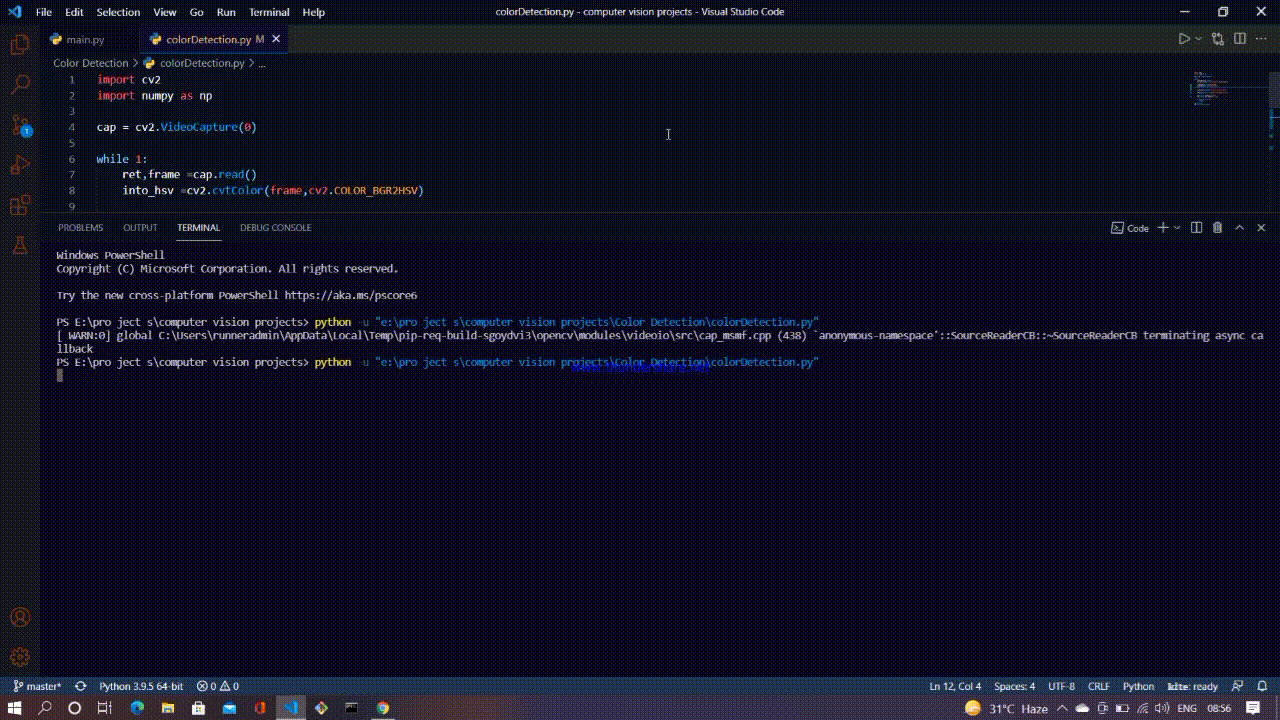Open in App
Not now

# Real time object color detection using OpenCV

• Last Updated : 03 Jan, 2023

In this article, we will discuss how to detect a monochromatic colour object using python and OpenCV. Monochromatic color means light of a single wavelength. We will use the video, captured using a webcam as input and try to detect objects of a single color, especially Blue. But you can detect any color if you set the range properly, we’ll discuss later.

To achieve that it’s highly recommended to convert the colors from RGB format to HSV.  HSV stands for hue, saturation, and value. Hue signifies the color itself, technically it denotes the angle in the HSV color model.

Let’s understand the HSV color model first. Imagine a cylinder, it has a height, a radius, and a curved surface area. Take the initial position of the radius as a reference and move the radius anticlockwise to an angle θ, i.e. θ is the angle between the current position of the radius with its initial position. If θ is 0 degrees then it denotes the color red, if θ is 120 degrees then it denotes green, and so on.  The length of the radius is the saturation of the color and the height signifies the value or V in HSV.

The main idea is to convert the input RGB image (BGR in the case of OpenCV because that’s how images are formatted in this module) to HSV format, which will make it easier for us to mask the specific color out of the frame. That is, whatever color in the provided HSV range will be given a value of 255 and others will be simply 0, and as a result, every object with color in the specified range will change to white leaving the rest of the image i.e. background black.

To show the color we need to bitwise and the current frame with the mask. For this, there is an inbuilt function called bitwise_and()

```syntax:

where pic1 and pic 2 are the input images, and the other is a mask. A mask can be thought of as a cut-out shape applied to an image so that only the cut-out part is visible.

### Implementation:

First, create an OpenCV video capture object after importing the required module using the following two codes:

```import cv2
import numpy as np```

Then start an infinite while loop, to read every frame read by the webcam. Convert every frame from BGR format to HSV format using the cv2.cvtColor() function, it takes the frame as the first input and the type of color conversion as the second input.

```syntax:
cv2.cvtColor(frame, cv2.COLOR_BGR2HSV)```

After that specify the lower and upper limit of the color blue ( or any color you prefer). By creating two NumPy arrays with the desired lower and upper limit as [H, S, V].  These two NumPy arrays will be used as arguments in the thresholding function i.e. the cv2.inRange() function. Which takes three arguments, image source, lower limit, and the upper limit.

```syntax:
cv2.inRange(source, lower_limit, upper_limit)```

cv2.inRange() function sets all the values that lie within the range to 255 and the rest to 0.  The output of this function will be our mask. Finally passing this mask in the bitwise_and function mentioned earlier will produce the desired result. The output is given below.

## Python3

 `import` `cv2``import` `numpy as np`` ` `cap ``=` `cv2.VideoCapture(``0``)`` ` `while` `1``:``    ``ret,frame ``=``cap.read() ``    ``# ret will return a true value if the frame exists otherwise False``    ``into_hsv ``=``cv2.cvtColor(frame,cv2.COLOR_BGR2HSV)``    ``# changing the color format from BGr to HSV ``    ``# This will be used to create the mask``    ``L_limit``=``np.array([``98``,``50``,``50``]) ``# setting the blue lower limit``    ``U_limit``=``np.array([``139``,``255``,``255``]) ``# setting the blue upper limit``        ` ` ` `    ``b_mask``=``cv2.inRange(into_hsv,L_limit,U_limit)``    ``# creating the mask using inRange() function``    ``# this will produce an image where the color of the objects``    ``# falling in the range will turn white and rest will be black``    ``blue``=``cv2.bitwise_and(frame,frame,mask``=``b_mask)``    ``# this will give the color to mask.``    ``cv2.imshow(``'Original'``,frame) ``# to display the original frame``    ``cv2.imshow(``'Blue Detector'``,blue) ``# to display the blue object output`` ` `    ``if` `cv2.waitKey(``1``)``=``=``27``:``        ``break``    ``# this function will be triggered when the ESC key is pressed``    ``# and the while loop will terminate and so will the program``cap.release()`` ` `cv2.destroyAllWindows()`

Output:My Personal Notes arrow_drop_up